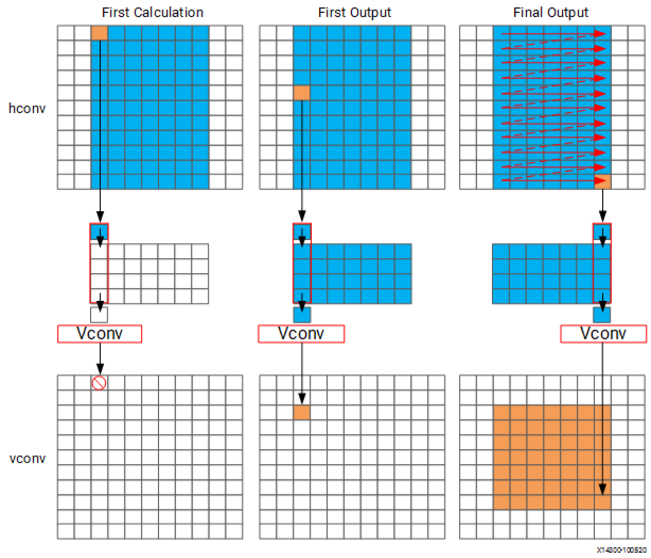Vertical Convolution - 2021.2 English

Vitis High-Level Synthesis User Guide (UG1399)

Document ID
UG1399
ft:locale
English (United States)
Release Date
2021-12-15
Version
2021.2 English

The vertical convolution represents a challenge to the streaming data model preferred by an FPGA. The data must be accessed by column but you do not wish to store the entire image. The solution is to use line buffers, as shown in the following figure.

Figure 1. Streaming Vertical ConvolutionOnce again, the samples are read in a streaming manner, this time from the hls::stream hconv. The algorithm requires at least K-1 lines of data before it can process the first sample. All the calculations performed before this are discarded.

A line buffer allows K-1 lines of data to be stored. Each time a new sample is read, another sample is pushed out the line buffer. An interesting point to note here is that the newest sample is used in the calculation and then the sample is stored into the line buffer and the old sample ejected out. This ensure only K-1 lines are required to be cached, rather than K lines. Although a line buffer does require multiple lines to be stored locally, the convolution kernel size K is always much less than the 1080 lines in a full video image.

The first calculation can be performed when the first sample on the Kth line is read. The algorithm then proceeds to output values until the final pixel is read.

// Vertical convolution
VConvH:for(int col = 0; col < height; col++) {
VConvW:for(int row = 0; row < vconv_xlim; row++) {
#pragma HLS DEPENDENCE variable=linebuf type=inter dependent=false
#pragma HLS PIPELINE